Naming Things
Formulas
Angle Relationships
Equations of Lines
Random
100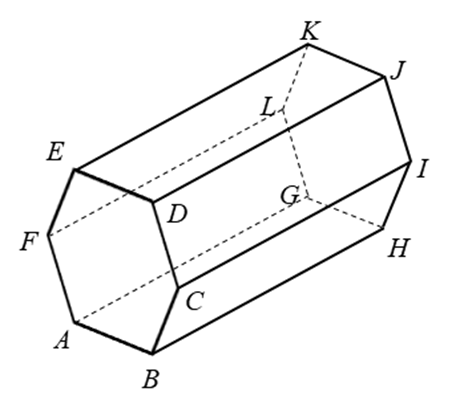Name a segment parallel to line segment AB

ED or GH

100
Parallel lines have what kind of slopes?

The same

100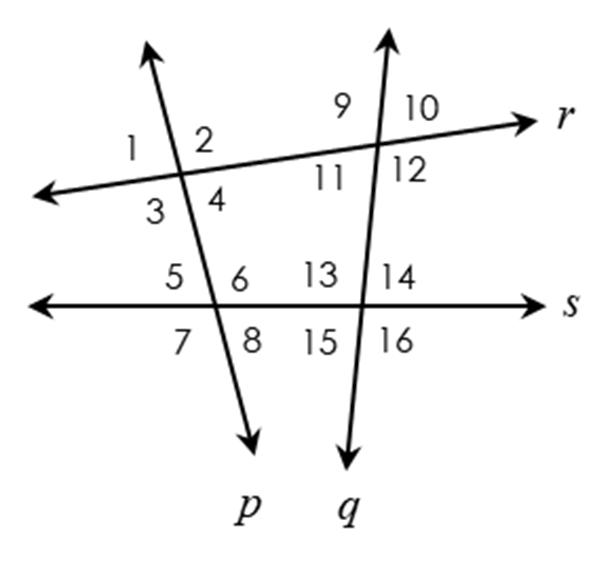What relationship are angles ∠9 and ∠16?

Alternate Interior

100

Determine whether QR and ST are parallel, perpendicular or neither.

Q(-1, 4), R(-1,  -9), S(11, -2), T(11, 6)

Parallel

100

What is the shape of a stop sign called?

octogan

200

Spin the wheel

Spin the wheel

200

Perpendicular lines have what kind of slope?

Negative reciprocal

200What relationship are angles ∠7 and ∠15

Corresponding

200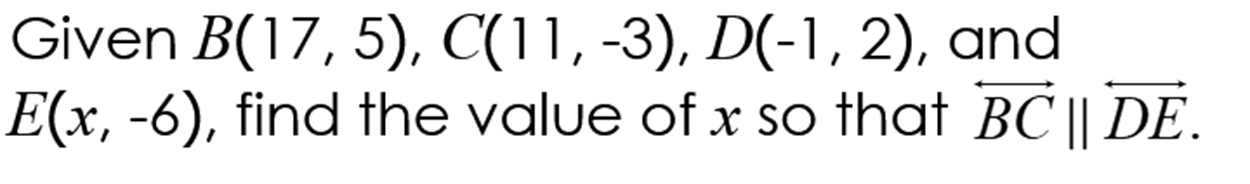200

Which country invented ice cream?

China

300Name a plane parallel to CIJ

300

What is slope intercept form?

y=mx+b

300

Spin the wheel!

Spin the wheel!

300

(RS) ̅ is formed by R(-2,-10) and S(10,-4). If line k is the perpendicular bisector of (RS) ̅ write an equation for k.

y=-2x-10

300
In what city was JFK assassinated in?

Dallas

400

Spin the Wheel!

400

Spin the Wheel!

Spin the wheel!

400

Find the value of x and state the converse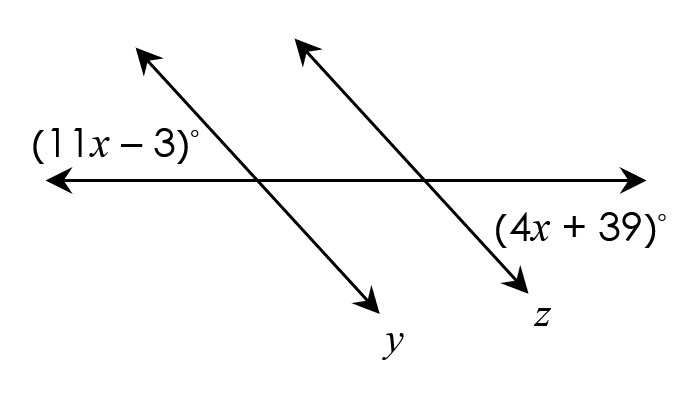400

Write a linear equation that passes through the point
(2, -9) and has a slope of -5 in slope-intercept form.

400

What soda was invented during World War 2

Fanta

500

Which segment is not skew to (EK) ̅?500

What is the slope formula?

y2-y1/x2-x1

500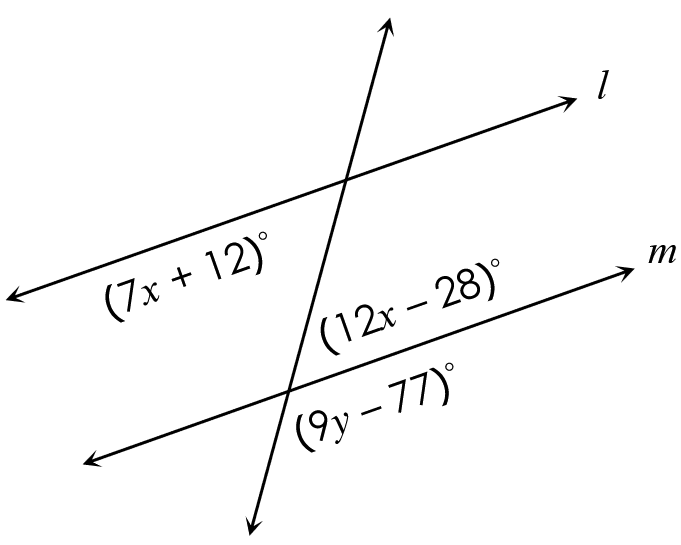Find the value of x and y

500

Spin the wheel

Spin the wheel

500

How many bones do sharks have?

None

600Which plane is parallel to ABC

JIH

600

What is the point-slope form?

y-y1=m(x-x1)

600What relationship are angles ∠3 and ∠5

600

Determine if QR and ST are parallel, perpendicular or neither.
Q(-6, 11), R(2, -1),
S(-4, 8), T(-1, 10)

600

In what area did algebra come from?

Middle East

Click to zoom